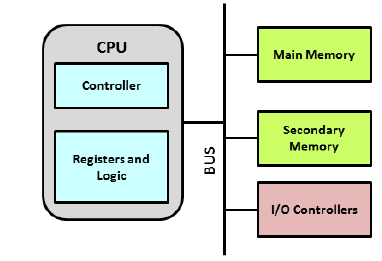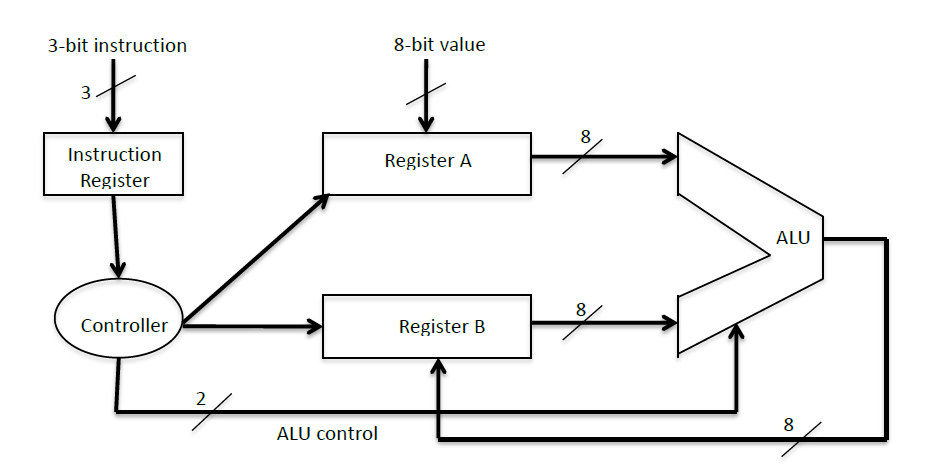# CSC 355 Lab 6: Very Small Computer System (VSCS) Solved

20.00 \$

Category:
Click Category Button to View Your Next Assignment | Homework

You'll get a: . ` zip` file solution, download link after Payment

## Description

### Introduction

The goal of this lab is to understand the design of a Simple Computer System and construct the circuit using Design Works. This Lab is entirely completed using Design Works.
An architectural block diagram of a simple computer is shown in Figure 1.
Figure 1This lab focuses on the design of the Central Processing Unit or CPU for a Very Small Computer System (VCVS.) The CPU contains:
all the internal registers;
the arithmetic and logic circuits;
the controller, which produces timing signals to coordinate the activities of the machine.
The workhorse of the Registers and Logic section of the CPU is the Arithmetic and Logic Unit (ALU). ALU circuits in larger computer systems contain additional circuitry for operations such as shifting and rotating sets of bits, multiplication and division and floating-point arithmetic. Our ALU here is more restricted in its selection of operations. Our ALU gets all of its operands from registers. The ALU circuit to use is provided on ConneX.
The CPU controls the execution of a sequence of instructions stored in a sequence in the main memory. A special register, the program counter (PC), stores the address of the beginning of the next instruction to be fetched and executed. The PC is updated as each instruction is executed. In this lab, we do not consider the storage of instructions in memory and the program counter. (But that is an excellent extension of this design!) We simulate that part of the CPU with a set of 3 input lines called INSTRUCTION that contain the 3-bit instruction as it would come from the memory.

### Specifications of the VCVS

The computer you are designing is an 8-bit accumulator architecture that takes a 3-bit instruction, decodes it and executes it. VCVS has two 8 bit registers (A and B) (use the REG-8 circuit in Design Works for these), an ALU and a 3-bit instruction register (use 3 bits of the REG-4 circuit in Design Works) as shown below in Figure 2. Note that not all control signals are shown in the diagram. The output of the ALU is always written back to the Register B (Figure 2).

### Lab 6: A Very Small Computer SystemFigure 2: Block Diagram of VCVS
The controller of the VCVS operates on an external clock signal. The VCVS takes three clock cycles (pulses) for every operation. In order to simplify our circuits, we will use 3 separate inputs X, Y and Z to simulate the three clock cycles, as shown in Figure 3:Figure 3
The VCVS controller operates as follows:
 In the first clock cycle, a 3-bit instruction is loaded (coming from binary switches) into the instruction register. The simulated control signal (X) will be used for this purpose.
 In the second clock cycle, the instruction is decoded and signals to perform the appropriate logic or arithmetic operation are asserted. The simulated control signal (Y) will be used for this:
o If the instruction is not CLR, then when signal Y goes high:
 Register A will be loaded from data found on binary switches, and
 the two bit ALU control signals (obtained from the instruction by the controller) is sent to the ALU.
o If the instruction is CLR, then when signal Y goes high: both register A and register B are cleared.
Controller
Register A
Instruction
Register
Register B
ALU
3-bit instruction
8-bit value
ALU control
2
3
8
8
8

Lab 6: A Very Small Computer System

 In the third clock cycle, the output of ALU is loaded back into register B. A simulated control signal (Z) is used for this:
o If the instruction is not CLR, then the ALU result is loaded into Register B.
o If the instruction is CLR, no action is taken (essentially, the ALU is disabled.)
The cycle repeats after this.
The format of the 3-bit instructions is specified as follows:
Bits
Mnemonic
Meaning
Use Design Works Feature:
000
B  B + A
001
SUBTR
B  B – A
two Subtractor-4
010
AND
B  B AND A
eight 2-input AND
011
OR
B  B OR A
eight 2-input OR
100
CLR
A  0; B  0
CLR input on Registers A and B
101
NOP
No operation
110
NOP
No operation
111
NOP
No operation
After decoding an instruction, there are three possible general categories of action:
1. If the decoded instruction is an arithmetic or logical operation (one of the first four), then the values that are in the two Registers (A and B) are be sent to the ALU for processing. The results from the ALU are always written back to the register B.
2. If the instruction is CLR, then registers A and B are cleared.
3. There are three opcodes, namely 101, 110 and 111,that are not used. These are simply specified as no operation (NOP) in the table. Note that when any of these instructions is present, the clock to the controller MUST be disabled so that no operation is performed. This provides future instruction expansion room for the system.
Pre -Lab ExerciseLab Exercise Lab Exercise Lab Exercise Lab Exercise Lab Exercise
1. Begin a new Generic Simulation in Design Works. Save your file with the name Lab6XXXX.cct where XXXX represents your surname.
2. On the circuit window place sufficient REG-8, REG-4, Adder-8, Subtractor-4, 2-input AND, and 2-input OR devices to realize the description below. Ensure they are groups neatly together so that connecting wires and additional devices can easily be connected. (There is no need for you to connect these devices at this stage. A connection block diagram will be provided in the lab period.)
3. Save your Lab6XXXX.cct file and submit it to the Pre-Lab 6 submission under the Assignments link on the course Connex site. (Submission deadline is 11:55 Friday October 28, 2016.)
In -Lab Exercises Lab Exercises Lab Exercises Lab Exercises Lab Exercises
1. Complete the Lab 6 tutorial (Lab6-tutorial.pdf) which is on the CSC 355 Connex site under resources/Labs/Lab6.
2. Locate the detailed block diagram of the VCVS CPU which is on the CSC 355 Connex site under resources/Labs/Lab6.
3. Make the connections and run a simulation of the execution of the Add, Subtract, AND, OR and clear instructions.